Mike on MATLAB GraphicsGraphics & Data Visualization

Note

Mike on MATLAB Graphics has been retired and will not be updated.

Interactive Graph Layout

I really like the new graph visualization functions that were introduced in R2015b. I particularly like the various options for laying out the graph so you can get a nice picture quickly and easily. But sometimes I like to be able to adjust the layout myself. The documentation for the graph functions don't provide much information about how to do this, but it's actually quite easy. Let's look at an example.

Here's a graph I really like. It comes from a problem called the square-pairs problem (although there's also another famous problem with that name involving primes). It creates nodes for several small integers, and then connects pairs of nodes with an edge if they sum to a square number.

function g = square_pairs(n)
s = [];
e = [];
for i=1:n
for j=(i+1):n
a = i + j;
q = round(sqrt(a));
if q^2 == a
s = [s, i];
e = [e, j];
end
end
end
g = graph(s,e);
g = square_pairs(24);
h = plot(g);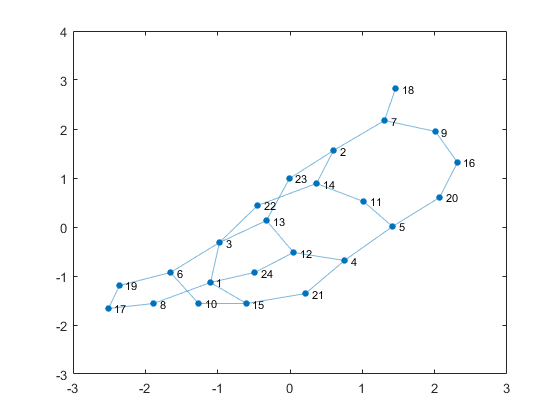The automatic layout does a pretty good job with that, but it could be better. I know that this graph is planar, but there are some edge crossings in this layout. I'd like to get rid of them. There are other layout options, such as 'circle' ...

layout(h,'circle')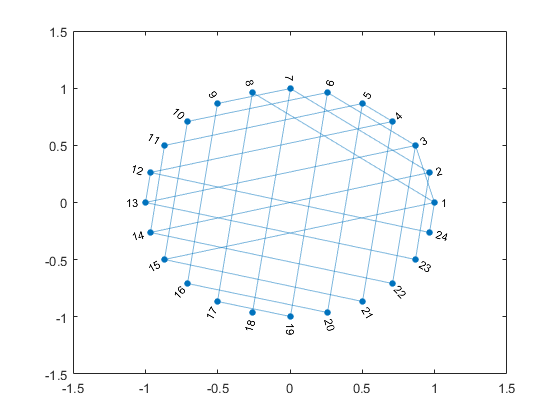... or layered.

layout(h,'layered')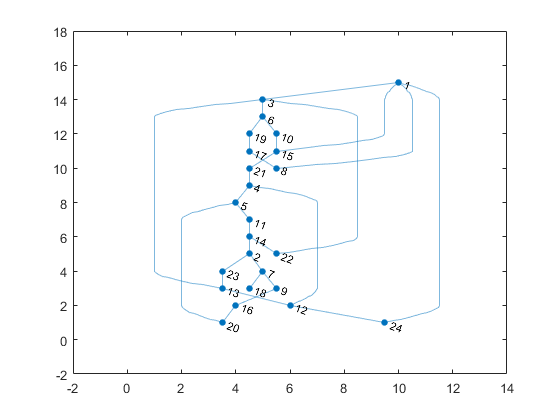But none of them are really better than the one that plot chose automatically.

layout(h,'auto')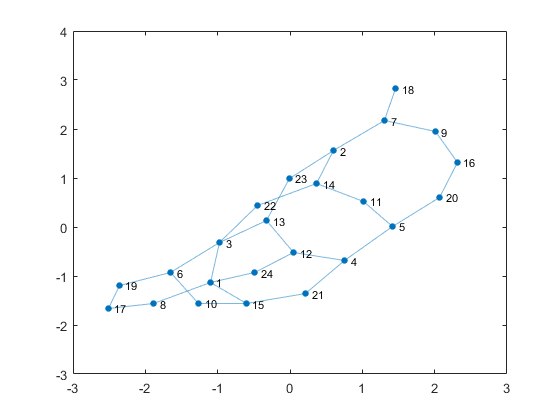If I want a better result, I'll have to lay the graph out by hand. I could do that by setting the XData and YData until I find values that look good, but I'd really like an interactive way to place the nodes.

It's actually pretty easy to do this. We'll just need to write a function that uses the position of the mouse to set the XData and YData. Let's walk through how I would write a function like this.

I'm going to call it edit_graph, and I'm going to use it like this:

set(gcf,'WindowButtonDownFcn',@(f,~)edit_graph(f,h))

The WindowButtonDownFcn is a property on each of the figure. If you set that to a function handle, the function will get called everytime you click on that figure. It takes two arguments, but we're only going to use the first one. That's a handle to the figure we've clicked on. We'll also need a handle to the graphplot object, so I pass that into edit_graph as a second argument.

The first thing we need to do is figure out where we've clicked. That's stored in a property on the axes named CurrentPoint. We can use the ancestor function to get a handle to the axes.

a = ancestor(e.Source,'axes');
pt = a.CurrentPoint(1,1:2);

Now we need to find the node in the graph that is closest to where we've clicked. That's the one we're going to move.

dy = h.YData - pt(2);
len = sqrt(dx.^2 + dy.^2);
[lmin,idx] = min(len);

Now lmin is the distance from where I clicked to the nearest node, and idx is the index of that node.

Next we check lmin. If it's large, then we didn't click near a node at all. In that case, we don't want to do anything.

tol = max(diff(a.XLim),diff(a.YLim))/20;
if lmin > tol || isempty(idx)
return
end

Otherwise we can just use idx as the node. We do need to worry about the case where the mouse is equidistant from multiple nodes, so we'll use idx(1) to grab the first of these.

node = idx(1);

Now we're ready to start moving the node!

To do that, we'll need to add two more callback functions to the figure. One will get called each time the mouse moves to a new position. That's called WindowButtonMotionFcn, and it's the one which will actually change the graph. The other function will get called when I let go of the mouse button. It's called WindowButtonUpFcn, and we'll use that to turn the other one off.

f.WindowButtonMotionFcn = @motion_fcn;
f.WindowButtonUpFcn = @release_fcn;

I'm going to do these as nested functions. That means that I'm going to declare them inside the edit_graph function. That will make it easy for them to share information.

Let's write the harder one first. It looks like this:

function motion_fcn(~,~)
newx = a.CurrentPoint(1,1);
newy = a.CurrentPoint(1,2);
h.XData(node) = newx;
h.YData(node) = newy;
drawnow;
end

The first two lines get the current location of the mouse. The next two lines stick those values into the XData and YData arrays at the index we found earlier. Then we call drawnow to tell the graphics system that we'd like to see the result of this change.

And then we just need to write release_fcn. That's pretty easy. It just sets these two function handles to empty.

function release_fcn(~,~)
f.WindowButtonMotionFcn = [];
f.WindowButtonUpFcn = [];
end

And that's it. Now I can start dragging the nodes around to adjust the layout. Here's what I came up with.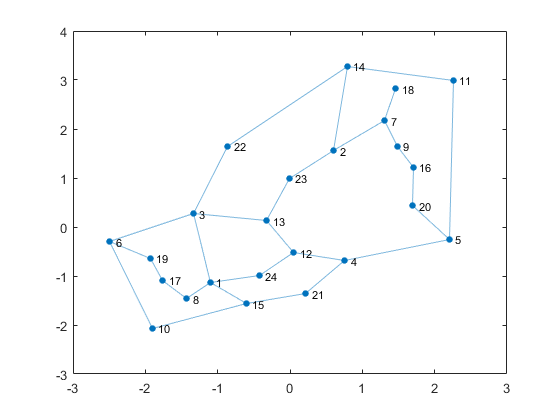You can get the completed edit_graph function here if you'd like to try to create a better layout of this graph. You could also use it to see if square_pairs(30) is also planar. And of course, you could use it to fiddle the layout of one of your own graphs.

Published with MATLAB® R2015b